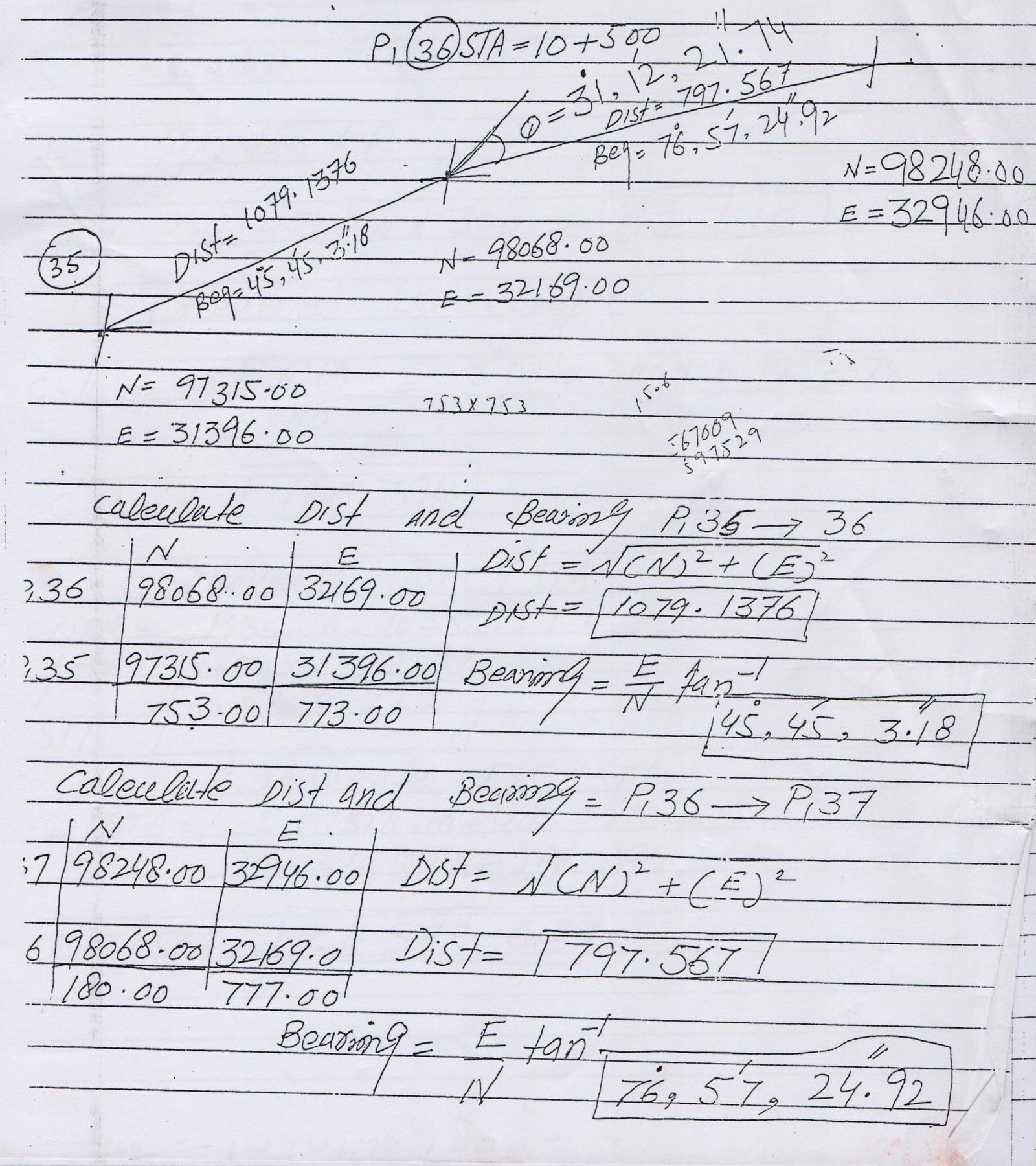# How to Calculate Distance and Bearing between Coordinates

## Calculate Distance and Angle/Bearing Between Two Coordinates

The distance between any two COORDINATES is the length of the line section joining the POINTS. There is just one line going through two COORDINATES. Thus, the distance between two COORDINATES can be determined by observing the length of this line portion associating the two POINTS.

 P. No. North East 35 98068.00 32169.00 36 97315.00 31396.00